3d Printing: Tetrahedron
The tetrahedron is a dual polyhedron of the tetrahedron

The objective of this series is to show different small vertices. We will use them to build large scheletons of polyhedra.

The first one is the tetrahedron: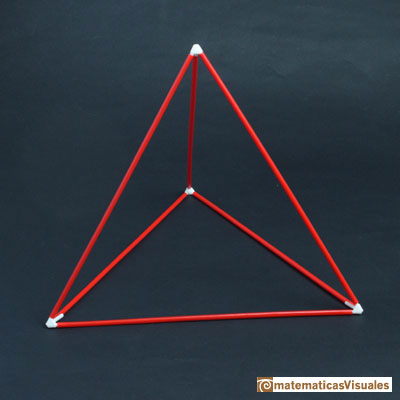To model these vertices I used OpenSCAD, a free wonderful program.The center of a tetrahedron

It is interesting to consider the center of the tetrahedron (in this case, the barycenter, the incenter and the circumcenter are the same point).Some molecules resemble this structure.

To model the central piece I used OpenSCAD: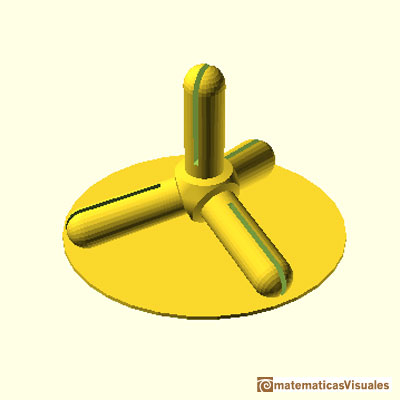This is the central piece and the four vertices printed:There are several way to calculate the position of the center. Here we are only remembering the main results. One way starts with the volume of the tetrahedron:The volume of a tetrahedron is one third of the prism that contains it.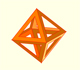The volume of an octahedron is four times the volume of a tetrahedron. It is easy to calculate and then we can get the volume of a tetrahedron.

Then the height of a regular tetrahedron is:

The center is on the height. The distance between one vertex and the center is:

This distance is the same as the circumradius: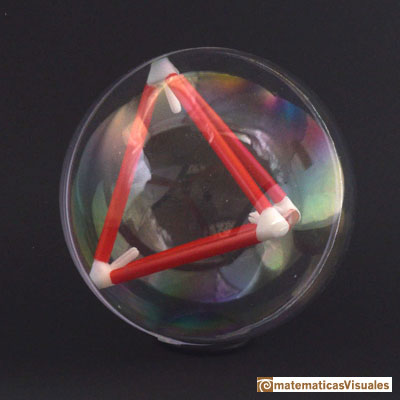The tetrahedron is a dual polyhedron of the tetrahedron

For every polyhedron there exists another polyhedron that is known as the dual or reciprocal. The vertices of one correspond with the faces of the dual polyhedron and vice versa.

We can see that the tetrahedron has the same number of vertices than faces. The dual of a tetrahedron is another tetrahedron. We say that the tetrahedron is self-dual.

One way to contruct a dual polyhedron of a regular polyhedron is to choose the center of the faces and connect each point with the points of its neighboring faces.

Then the centers of the faces of a tetrahedron form another tetrahedron.This construction was well known. Johannes Kepler published this illustration in his book "Harmonices Mundi" in 1619.It is not difficult to calculate the lenght of the edge of the small tetrahedron. Here we can consider a relation between the Circumradius and the Inradius of these polyhedra:

The circumradius of the small tetrahedron should be equal to the inradius of the bigger one:Then: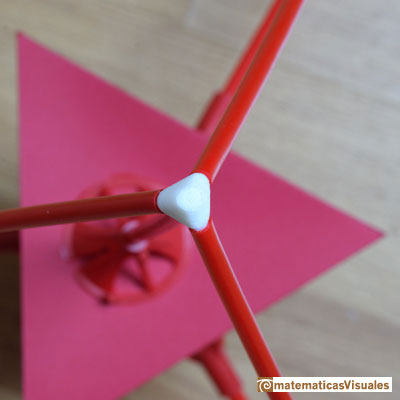There is an easy way to see the relation between the sides of the big tetrahedron and the small one. See this picture: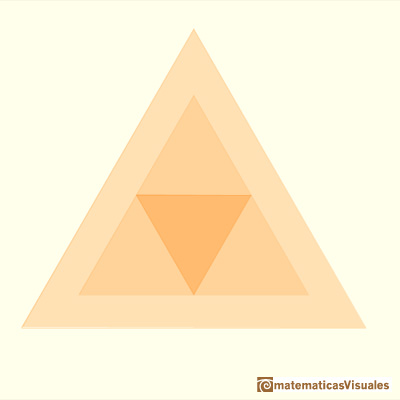The three vertices of the triangle on the middle are three barycenters of three faces of the big tetrahedron.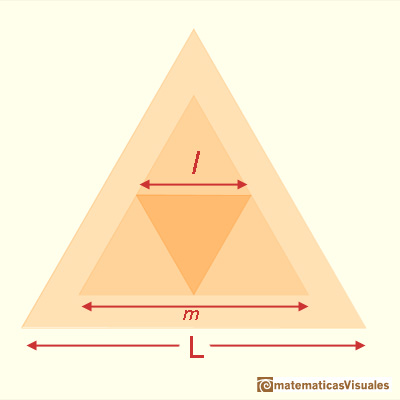Then,

REFERENCES

OpenSCAD a free wonderful program to model shapes in three dimensions.
Magnus Wenninger - 'Polyhedron Models', Cambridge University Press.
Hugo Steinhaus - Mathematical Snapshots - Oxford University Press - Third Edition.
Peter R. Cromwell - 'Polyhedra', Cambridge University Press, 1999.
H.Martin Cundy and A.P. Rollet, 'Mathematical Models', Oxford University Press, Second Edition, 1961.
W.W. Rouse Ball and H.S.M. Coxeter - 'Matematical Recreations & Essays', The MacMillan Company, 1947.Using cardboard you can build beautiful polyhedra cutting polygons and glue them toghether. This is a very simple and effective technique. You can download several templates. Then print, cut and glue: very easy!Material for a session about polyhedra (Zaragoza, 9th May 2014). Simple techniques to build polyhedra like the tetrahedron, octahedron, the cuboctahedron and the rhombic dodecahedron. We can build a box that is a rhombic dodecahedron.Material for a session about polyhedra (Zaragoza, 7th November 2014). We study the octahedron and the tetrahedron and their volumes. The truncated octahedron helps us to this task. We build a cubic box with cardboard and an origami tetrahedron.Material for a session about polyhedra (Zaragoza, 23rd Octuber 2015) . Building a cube with cardboard and an origami octahedron.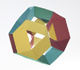Material for a session about polyhedra (Zaragoza, 21st October 2016). Instructions to build several geometric bodies.Italian designer Bruno Munari conceived 'Acona Biconbi' as a work of sculpture. It is also a beautiful game to play with colors and shapes.Microarquitectura is a construction game developed by Sara San Gregorio. You can play and build a lot of structures modelled on polyhedra.The volume of a tetrahedron is one third of the prism that contains it.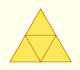The first drawing of a plane net of a regular tetrahedron was published by Dürer in his book 'Underweysung der Messung' ('Four Books of Measurement'), published in 1525 .In his article 'Two Surprising Theorems on Cavalieri Congruence' Howard Eves describes an interesting tetrahedron. In this page we calculate its cross-section areas and its volume.We want to calculate the surface area of sections of a sphere using the Pythagorean Theorem. We also study the relation with the Geometric Mean and the Right Triangle Altitude Theorem.Howard Eves's tetrahedron is Cavalieri congruent with a given sphere. You can see that corresponding sections have the same area. Then the volumen of the sphere is the same as the volume of the tetrahedron. And we know how to calculate this volumen.Special sections of a tetrahedron are rectangles (and even squares). We can calculate the area of these cross-sections.The truncated tetrahedron is an Archimedean solid made by 4 triangles and 4 hexagons.The twelve vertices of an icosahedron lie in three golden rectangles. Then we can calculate the volume of an icosahedronSome properties of this platonic solid and how it is related to the golden ratio. Constructing dodecahedra using different techniques.The first drawing of a plane net of a regular dodecahedron was published by Dürer in his book 'Underweysung der Messung' ('Four Books of Measurement'), published in 1525 .The first drawing of a plane net of a regular octahedron was published by Dürer in his book 'Underweysung der Messung' ('Four Books of Measurement'), published in 1525 .